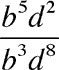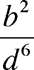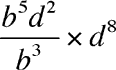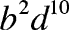Quandaries and Queries Chris 9th grade Parent Simplifying Exponents Question b^5d^2/b^3d^8 my answer b^3d^6 calculator b^3d^10 My daughter and I do not understand why. Thank you Hi Chris, I think the problem might be interpreting this expression without parentheses. When I looked at your expression b^5d^2/b^3d^8 I read is asIf this is the correct interpretation then it simplifies toIf the problem isThen the simplification isDoes this help? Penny Go to Math Central